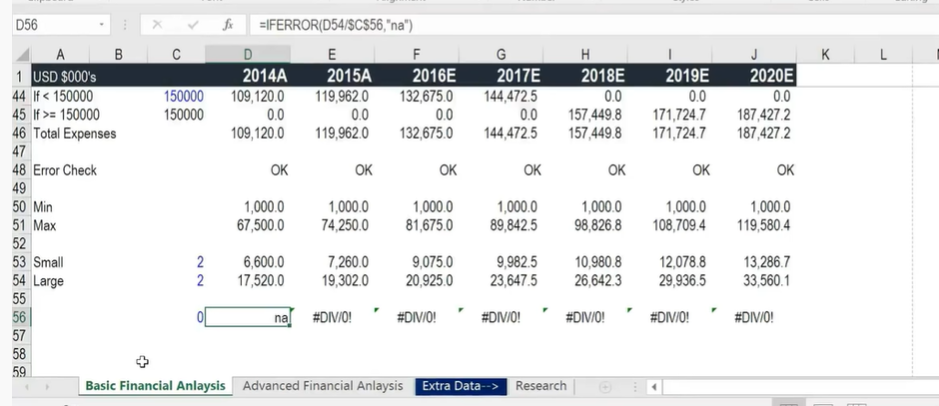# Excel Crash Course for Finance Professionals — FREE | Corporate Finance Institute

1. if you want to paste the formula to right of the cells, select the cells with the formula and ‘shift’ press right and ctrl+ ‘R’.

2. Format cells

select the cells you want to format and press ctrl + ‘1’

3. To add a letter behind a value but excel still treat it as value

type: 0"what you want to add”

4. change the background colour of selected cells

select the cells you want to change the background colour. Press ‘alt’ + h + h and use the arrow key to choose colour.

5. press ‘alt’ will show you all the shortcuts

6. ctrl + space will select the entire column

7. change width of column

alt + h + o + w

8. financial information are usually in landscape format

9. freeze pane

alt + w + f +f

10. bold

ctrl + b

11. change worksheet

ctrl + page up/down

12. press f2 to check the formula of a cell

13. use a different colour to differentiate between hardcoded numbers and formulated numbers. easier for person checker your work

14. decimal place

alt + h + 0 → increase decimal place

alt + h + 9 → decrease decimal place

15. borders

alt + h + b

16. grid lines

alt + w + vg

## Basic formulas and functions

1. Dates and Time

=today() → this date will get updated every day

you need to hard code the date if you want to keep it the same

=eomonth(reference to the current date, plus how many months) → end of month

=yearfrac()

2. Sum, average, sumproduct

to sum up the figures above, press alt + =

=average()

=sumproduct()

=median()

3. if statement

=if()

to align, press alt + h + ar

=”if ≥” & C45 → this is a dynamic formula as if C45 is changed, A45 will display the updated changes. *press f2 to choose a cell

4. Rounding and absolute references

=round()

=mround() →multiple rounding.

=ABS()

5. Min, Max, large and small tools

=min()

=max()

=small(array, nth smallest)

=large(array, nth largest)

6. Iferror, find and replace

=iferror() → if there is one error, it does not affect your outputfor instance, there will be an error if divide by ‘0’. so we replace the error message

press ctrl + f to go to find.

find and replace formulas are very useful.

***************************The end**************************

--

--